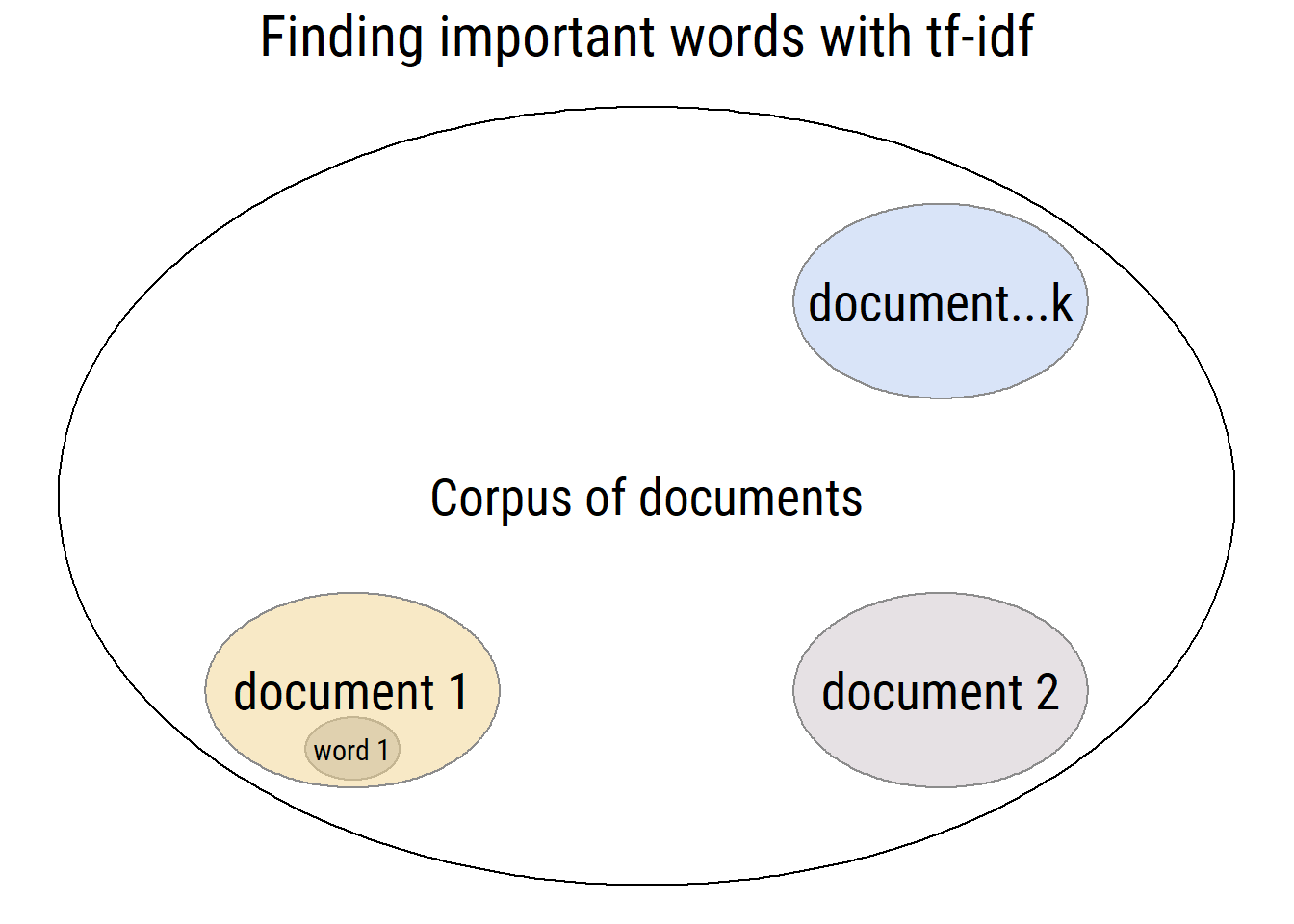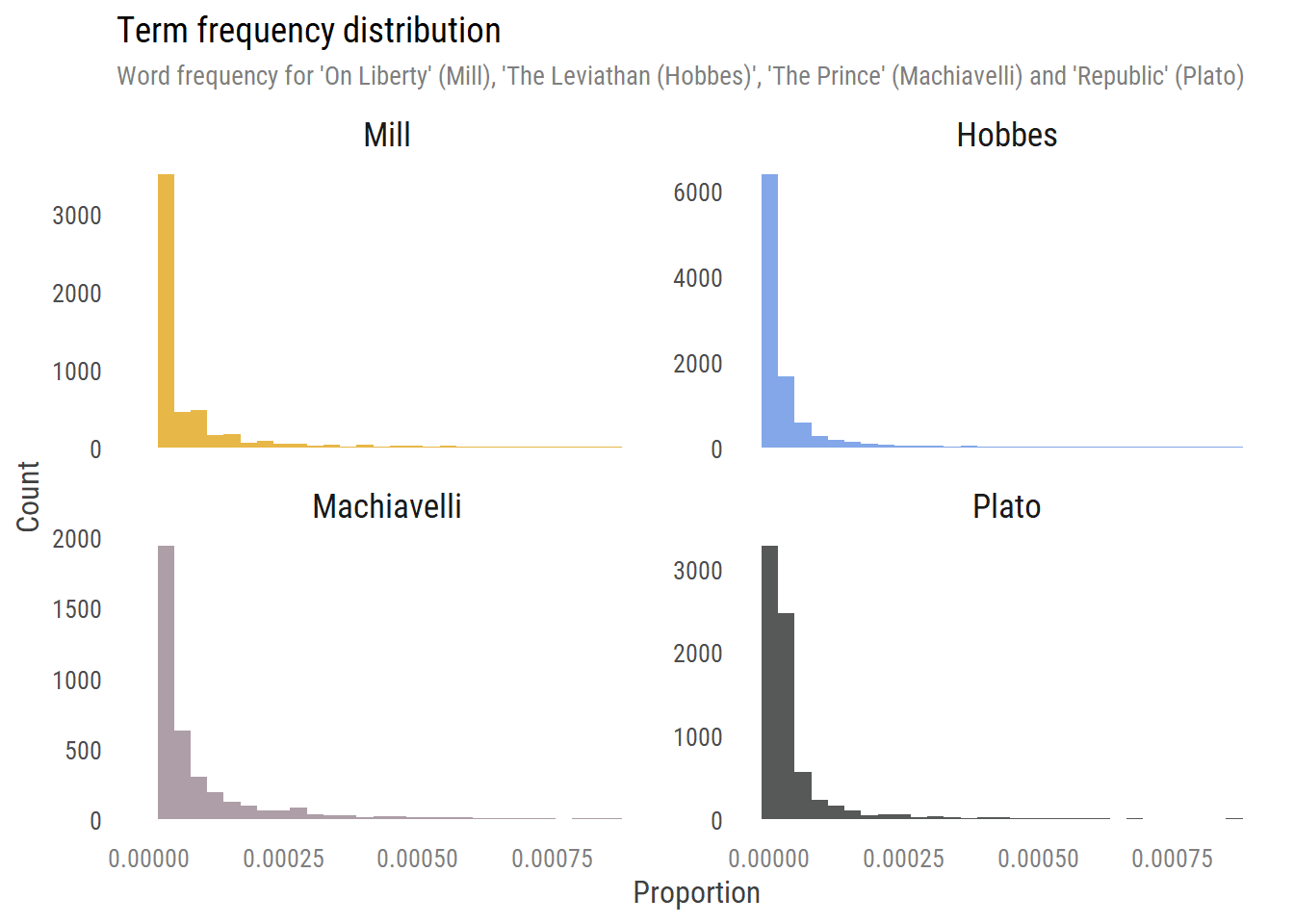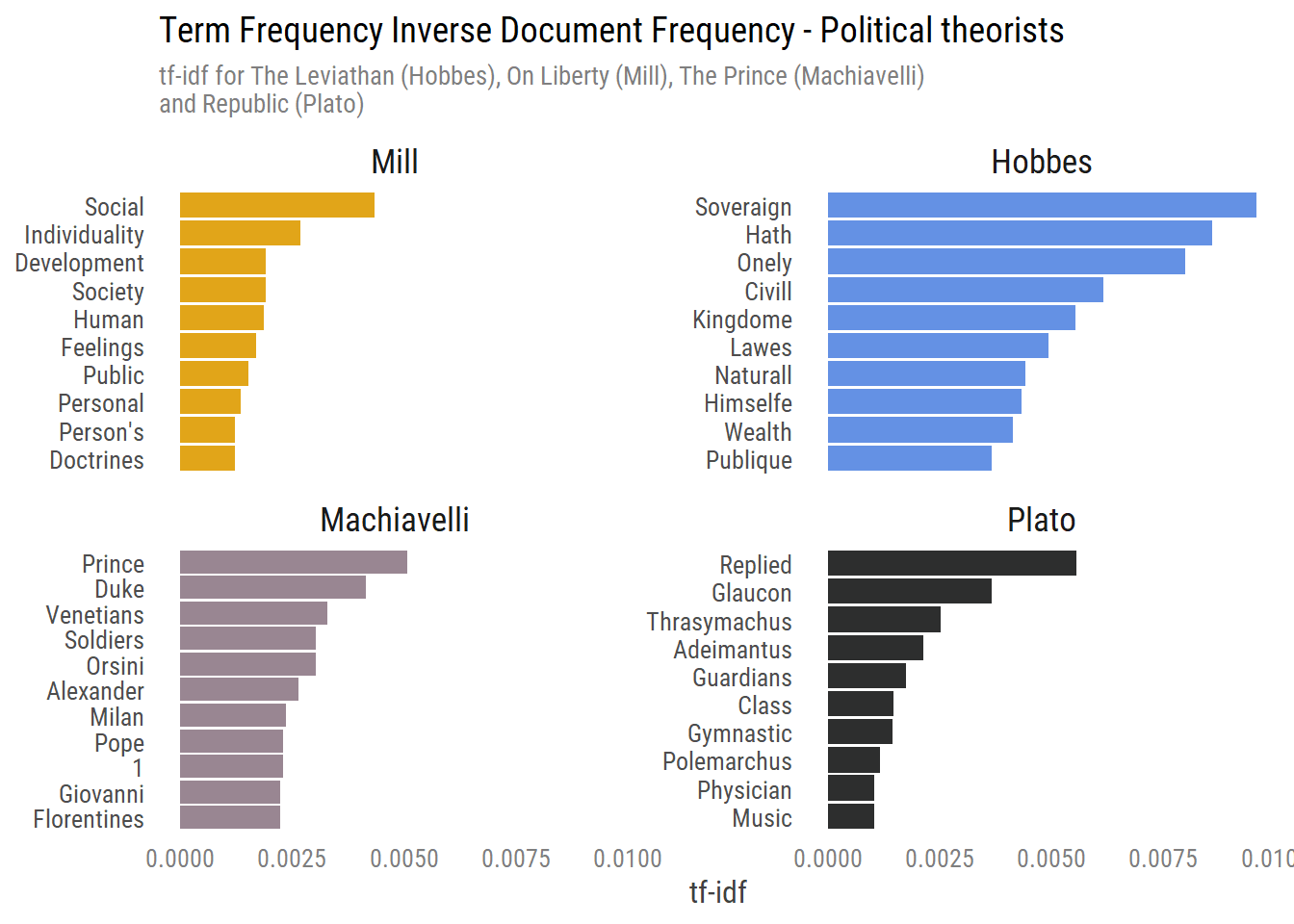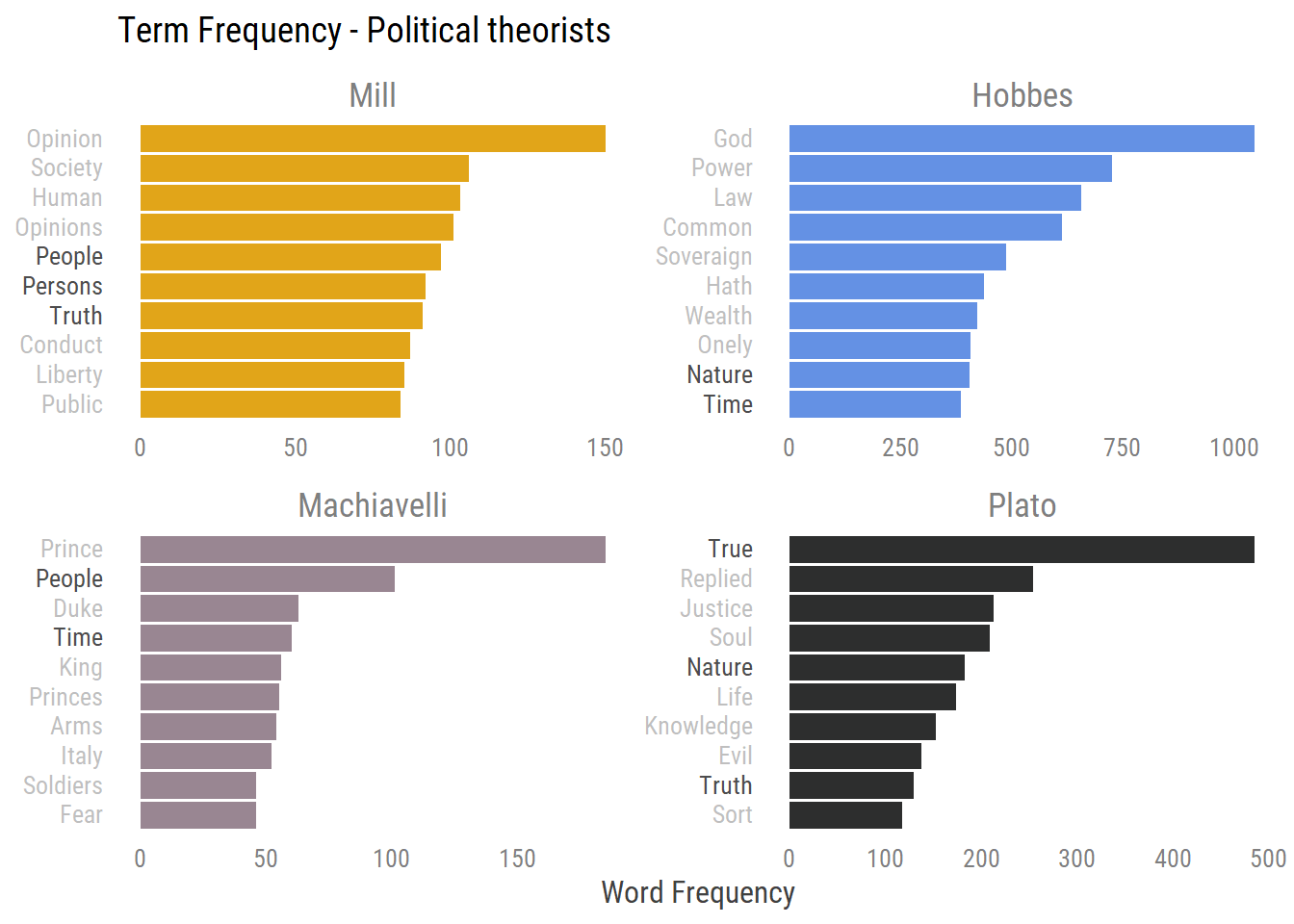Thanks to Almog Simchon for insightful comments on a first draft of this post.

### Introduction

Learning R for the past nine months or so has enabled me to explore new topics that are of interest to me, one of them being text analysis. In this post I’ll explain what is Term-Frequency Inverse Document Frequency (tf-idf) and how it can help us explore important words for a document within a corpus of documents1. The analysis helps in finding words that are common in a given document but are rare across all other documents.

Following the explanation we’ll implement the method on four great philosophers' books: ‘Republic’ (Plato), ‘The Prince’ (Machiavelli), ‘Leviathan’ (Hobbes) and lastly, one of my favorite books - ‘On Liberty’ (Mill) 😍. Lastly, we’ll see how tf-idf compares to a Bag of Words analysis (word count) and how using both can benefit your exploring of text.

The post is aimed for anyone exploring text-analysis and wants to learn about tf-idf. I will be using R to analyze our data but won’t be explaining the different functions, as this post focuses on the tf-idf analysis. If you wish to see the code, feel free to download or explore the .Rmd source code on my github repository.

## Term frequency

tf-idf gauges a word’s value according to two parameters: The first parameter is the term-frequency of a word: How common is a word in a given document (Bag of Words analysis); one method to calculate term frequency of a word is just to count the total number of times each words appears. Another method - which we’ll use in the tf-idf - is, after summing the total number of times a word appears, we’ll divide it by the total number of words in that document, describing term frequency as such:

$$tf = \frac{\textrm{N times a word appears in a document}}{\textrm{Total words in that document}}$$

Also written as $$tf(t,d)$$ where $$t$$ is the number of times a term appears out of all words in document $$d$$. Using the above method we’ll have the proportion of each word in our document, a value ranging from 0 to 1, where common words will have higher values.

While this gives us a value gauging how common a word is in a document, what happens when we have many words across many documents? How do we find unique words for each document? This brings us to idf.

## Inverse document frequency

Inverse document frequency accounts for the occurrence of a word across all documents, thereby giving a higher value to words appearing in less documents. In this case, for each term we will calculate the log ratio2 of all documents divided by the number of documents that word appears in. This gives us the following:

$$idf = \log {\frac{\textrm{N documents in corpus}}{\textrm{n documents containing the term}}}$$

Also written as $$idf = \log{\frac{N}{n(t)}}$$ Where $$N$$ is the total number of documents in our corpus and $$n(t)$$ is the number of documents the word appears within our corpus of documents.

To those unfamiliar, a logarithmic transformation helps in reducing wide-ranged numbers to smaller scopes. In this case, if we have 7 documents, and our term appears in all 7 documents, we’ll have following idf value: $$log_e(\frac{7}{7}) = 0$$. What if we have a term that appears in only 1 document out of all 7 documents? We’ll have the following: $$log_e(\frac{7}{1}) = 1.945$$. Even if a word appears in only 1 document out of 100, a logarithmic transformation will reduce its high value to mitigate bias when we multiply it with its $$tf$$ value.

So what do we understand from the idf? Since our numerate always remains the same (N documents in corpus), the idf of a word is contingent upon how common it is across documents. Words that appear in a small number of documents will have a higher idf, while words that are common across documents will have a lower idf.

## Term-Frequency Inverse Document Frequency (tfidf)

Once we have the term frequency and inverse document frequency for each word we can calculate the tf-idf by multiplying the two: $$tf(t,d) \cdot idf(t,D)$$ where $$D$$ is our corpus of documents.

To summarize our explanation: The two paramteres used to calculate the tf-idf provide each word with a value for its importance to that document in that corpus of text. Ideally We take words that are common within a document and that are rare across documents. I write ideally because as we’ll see soon, we might have words that are extremely common in one document but are filtered out because they’re evident in all documents (can happen in a small corpus of documents). This also highlights the question as to what is important; I define important as contributing to understanding a document in comparison to all other documents.Figure 1: Using tf-idf we can calculate how common a word is within a document and how rare is it across documents

Now that we have some background as to how tf-idf works, let’s dive in to our case study.

## TF-IDF on political theorists.

I’m a big fan of political theory. I have a small collection at home and always like to read and learn more about it. Except for Mill, we read Plato, Machiavelli and Hobbes in our BA first semester course in political theory. While some of the theorists overlap to some degree, over-all they discuss different topics. tf-idf will help us distinguish important words specific to each book, in a comparison across all books.

Before we conduct our tf-idf we’d like to explore our text a bit. The following exploratory analysis is inspired from Julia Silge’s blog post ‘Term Frequency and tf-idf Using Tidy Data Principles’, a fantastic read.

## Data collection & Analysis

The package we’ll use to gather the data is the {gutenbergr} package. It enables us to access the Project Gutenberg free books, a library of over 60,000 free books. As many other amazing things in R someone, in this case David Robinson, created a package for it. All we need to do is download them to our computer.

Mill <- gutenberg_download(34901)


Several of the books contain sections at the beginning or at the end that aren’t relevant for our analysis. For example long introductions from contemporary scholars; another whole different book at the end, etc. These can confound our analysis and therefore we’ll exclude them. In order to conduct our analysis we also need all the books we collected in one object.

Once we are able to clean the books, this is what our text looks like:

remove_text <- function(book, low_id, top_id = max(rowid), author = deparse(substitute(book))){
book %>%
mutate(author = as.factor(author)) %>%
rowid_to_column() %>%
filter(rowid >= {{low_id}}, rowid <= {{top_id}}) %>%
select(author, text, -c(rowid, gutenberg_id))}

books <- rbind(
remove_text(Mill, 454),
remove_text(Hobbes, 360, 22317),
remove_text(Machiavelli, 464, 3790),
remove_text(Plato, 606))

## # A tibble: 45,490 × 2
##    author text
##    <fct>  <chr>
##  1 Mill   ""
##  2 Mill   ""
##  3 Mill   "CHAPTER I."
##  4 Mill   ""
##  5 Mill   "INTRODUCTORY."
##  6 Mill   ""
##  7 Mill   ""
##  8 Mill   "The subject of this Essay is not the so-called Liberty of the Will, …
##  9 Mill   "unfortunately opposed to the misnamed doctrine of Philosophical"
## 10 Mill   "Necessity; but Civil, or Social Liberty: the nature and limits of th…
## # … with 45,480 more rows


Each row is some text with chapters separated by headings and a column referencing who is the author. Our data frame consists of ~45,000 rows with the filtered text from our four books. Tf-idf can also be done on any n-grams we choose (number of consequent words). We could calculate the tf-idf for each bigram of words (two-words), trigram, etc. I find a unigram an appropriate approach both for tf-idf and especially now when we want to learn more about it. We just saw that our text is in the form of sentences, so let’s break it into single words.

## # A tibble: 12 × 4
## # Groups:   author 
##    author      word      n sum_words
##    <fct>       <chr> <int>     <int>
##  1 Hobbes      the   14570    208341
##  2 Hobbes      of    10550    208341
##  3 Hobbes      and    7144    208341
##  4 Plato       the    7054    118639
##  5 Plato       and    5746    118639
##  6 Plato       of     4640    118639
##  7 Mill        the    3019     48006
##  8 Mill        of     2461     48006
##  9 Machiavelli the    1923     34108
## 10 Mill        to     1765     48006
## 11 Machiavelli to     1452     34108
## 12 Machiavelli and    1288     34108


We see that stop-words dominant the frequency of occurrences (second column. That makes sense as they are commonly used, but they’re not usually helpful for learning about a text, specifically here. We’ll start by exploring how the word frequencies occur within a text:The plot above shows the frequency of terms across documents. We see some words that appear frequently (higher proportion = right side of the x-axis) and many words that are rarer (low proportion). Actually, I had to limit the x-axis or otherwise it would distort the plot with words that are extremely common.

To help find useful words with the highest tf-idf from each book, we’ll remove stop words before we extract the words with a high tf-idf value:

Author Word n Sum words Term Frequency IDF TF-IDF
Mill opinion 150 48006 0.0094132 0.0000000 0.0000000
Hobbes god 1047 208341 0.0148665 0.0000000 0.0000000
Machiavelli prince 185 34108 0.0176040 0.2876821 0.0050643
Plato true 485 118639 0.0152953 0.0000000 0.0000000

Random sample of words and their corresponding tf-idf values

Above we have our tf-idf for a given word from each document. I removed stop-words and calculated the tf-idf for each word in each book. For Hobbes the word ‘God’ appears 1047 times, thus has a $$tf$$ of $$\frac {1047} {207849}$$ and an idf of 0 (since it appears in all documents), so it’ll have a tf-idf of 0.

With Machiavelli the word prince appears 185 times, with a $$tf$$ of $$\frac {185} {34821}$$, resulting in a proportion of 0.0173. The word prince has an idf of 0.288 $$(log_e(\frac 4 {3}))$$, as there are 4 documents and it appears in 3 of them, so a total tf-idf value of $$0.0173 \cdot 0.288$$ = $$0.00497$$.

## Tf-idf plot

As we wrap up our tf-idf analysis, We don’t want to see all words and their tf-idf, but only words with the highest tf-idf value for each author, indicating the importance of a word to a given document. We can look at these words by plotting the top 10 highest valued tf-idf words for each author:

 ggplot(data = books_for_plot, aes(x = word, y = tf_idf, fill = author))+
geom_col(show.legend = FALSE)+
labs(x = NULL, y = "tf-idf")+
coord_flip()+
scale_x_reordered()+
facet_wrap(~ author, scales = "free_y", ncol = 2)+
labs(title = "<b>Term Frequency Inverse Document Frequency</b> - Political theorists",
subtitle = "tf-idf for The Leviathan (Hobbes), On Liberty (Mill), The Prince (Machiavelli)\nand Republic (Plato)")+
scale_fill_manual(values = plot_colors)+
theme_post+
theme(plot.title = element_markdown())Lovely!

Let’s review each book and see what we can learn from our tf-idf analysis. My memory of these books is kind of rusty but I’ll try my best:

• Hobbes: Hobbes in his book describes the natural state of human beings and how they can leave it by revoking many of their right to the sovereign who will facilitate order. In his book he describes the soveragin (note the ‘a’) as needed to be strict, rigorous and hath.

• Machiavelli: Machiavelli provides a leader with a guide on how to rule his country. He prefaces his book with an introduction letter to the Duke, the recipient of his work. Machiavelli throughout the book conveys his message with examples of many princes, Alexander the great, the Orsini brothers and more. Several of his examples include mentioning of Italy (where he resides), specifically Venetians and Milan.

• Mill: Mill in his book ‘On Liberty’ describes the importance of freedom and liberty for individuals. He does so by describing the relation between people and their society and other relations with the social. He highlights in his discussion on liberty a person’s belonging; these can be Feelings or basically anything personal. Protecting the personal is important for the development of both society and that of the individual.

• Plato: Plato’s book consists of 10 chapters and it is by far the longest compared to the others. The book is written in the form of a dialogue with replies between Socrate and his discussants. Along Socrate’s journey to finding out what is the meaning of justice he talks to many people, among them Glaucon, Thrasymachus and Adeimantus. In one section Socrates describes a just society with distinct classes such as the guardians. The classes should receive appropriate education, for e.g. gymnastics for the guardians.

With the above analysis we were able to explore uniqueness of words for each book across all books. Some words provided us with great insights while others didn’t necessarily help us despite their uniqeness, for example, the names of discussants with Socrate. Tf-idf gauges them as important (as to how I defined importance here) to distinguish between Plato’s book and the others, but I’m sure they’re not the first words that come to mind when someone talks about the Republic.

The analysis also shows this methodology’s value addition is not in just applying tf-idf - or any other statistical analysis – rather its power lies in its explanatory abilities. In other words, tf-idf provides us with a value indicating the importance of a word to a given document within a corpus, it is our job to take that extra step interpreting and contextualizing the output.

## Comparing to Bag Of Words (BOG)

A common text analysis is a word count I discussed earlier, also known as Bag of Words (BoW). This is an easy to understand method that can be done easily when exploring text. However, relying only on a bag of words method to draw insights can limit its usefulness if other analytic methods are not also included. The BoW relies only on the frequency of a word, so if a word is common across all documents, it might show up in all of them and not contribute to finding unique words for each document.

Now that we have our books we can also explore the raw occurrence of each word to compare it to our above tf-idf analysis:

ggplot(data = bow_books, aes(x = reorder(word_with_color,n), y = n, fill = author))+
geom_col(show.legend = FALSE)+
labs(x = NULL, y = "Word Frequency")+
coord_flip()+
scale_x_reordered()+
facet_wrap(~ author, scales = "free", ncol = 2)+
labs(title = "<b>Term Frequency</b> - Political theorists")+
scale_fill_manual(values = plot_colors)+
theme_post+
theme(axis.text.y = element_markdown(),
plot.title = element_markdown(),
strip.text = element_text(color = "grey50"))Figure 2: Term frequency plot with words that are common across documents in bold

The above plot amplifies, in my opinion, tf-idf’s contribution in finding unique words for each document. While many of the words are similar to those we found in the previous tf-idf analysis, we also draw words that are common across documents. For example, we see the frequency of ‘Time’, ‘People’ and ‘Nature’ twice in different books and words such as ‘True’ and ‘Truth’ with similar meanings do so too (however this could have happened in tf-idf too).

However, the Bag of Words also provided new words we didn’t see earlier. Here we can learn on new words like Power in Hobbes, Opinions in Mill and more. With the bag of words we get words that are common without controlling for other texts, while the tf-idf searches for words that are common within but are rare across.

## Closing remarks

In this post we learned the term frequency inverse document frequency (tf-idf) analysis and implemented it on four great political theorists. We finished by exploring tfidf in comparison to a bag of words analysis and showed the benefits of each. This also emphasizes how we define important: Important to a document by itself or important to a document compared to other documents. The definition of ‘important’ here also highlights tf-idf heuristic quantifying approach ( specifically the idf) and thus should be used with caution. If you are aware of theoretical development of it I’d be glad to read more about it.

By now you should be equipped to give tf-idf a try yourself on a corpus of documents you find appropriate.

### Where to next

• Further reading about text analysis - If you want to read more on text mining with R, I highly recommend the Julia Silge & David Robinson’s text mining with R bookand/or exploring the {quanteda} package.

• Text datasets - As to finding text data, you can try the {gutenbergr} package that gives access to thousands of books, a #TidyTuesday data set or collect tweets from Twitter using the {rtweet} package.

• Other posts of mine - If you’re interested in other posts of mine where I explore some text you can read my Israeli elections Twitter tweets analysis.

That’s it for now. Feel free to contact me for any and all comments!

1. A single document can be a book, chapter, paragraph or sentence, it all depends on your research and what you define as an ‘entity’ within a corpus of text. ↩︎

2. What’s log ratio? In general, and for the purpose of the tf-idf, a logarithm transformation (in short $$log$$) helps in reducing wide ranged numbers to smaller scopes. Assuming we have the following $$\log _{2}(16) = x$$, we ask ourselves (and calculate) 2 in the power of what (x) will give us 16. so in this case 2^3 will give us 16, which is basically written as $$\log _{2}(16) = 3$$. In order to generalize it, $$\log _{b}(x) = y$$, means b is the base we will raise to the power of y to reach x. Therefore written oppositely as $$b^y = x$$. The common uses of log are $$\log_2$$, $$\log_{10}$$ and $$log_e$$, also written as plain log. ↩︎